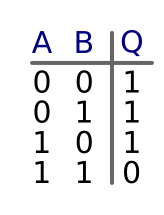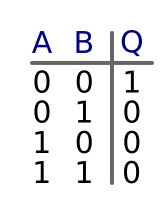# Inverting gates

Martin McBride, 2017-01-04
Tags not gate nand gate nor gate truth table
Categories logic logic gates

There are two main types of inverting gates - NAND and NOR gates.

## NAND gates

A NAND gate (NOT AND) is similar to an AND gate, but it produces the opposite output - if both inputs are 1, the output is 0, otherwise the output is 1.

This is the symbol for a NAND gate:You can think of the gate as being equivalent to an AND gate followed by a NOT gate:## NAND Truth table

Here is the truth table for a NAND gate:## NOR gates

A NOR gate (NOT OR) is similar to an OR gate, but it produces the opposite output - if either (or both) inputs are 1, the output is 0, otherwise the output is 1.

This is the symbol for a NOR gate:You can think of the gate as being equivalent to an OR gate followed by a NOT gate:## NOR Truth table

Here is the truth table for a NOR gate:## Video

Copyright (c) Axlesoft Ltd 2021